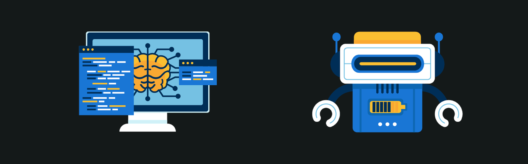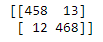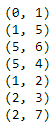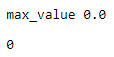# Latest Machine Learning Projects to Try in 2019Kurt is a Big Data and Data Science Expert, working as a...

Machine Learning is clearly a field that has seen crazy advancements in the past couple of years. This trend and advancements have created a lot of Job opportunities in the industry. The need for Machine Learning Engineers are high in demand and this surge is due to evolving technology and generation of huge amounts of data aka Big Data. So, in this article, I’ll be discussing the most amazing Machine Learning Projects one should definitely know and work with, in the following order:

## What is Machine Learning?

Machine Learning is a concept which allows the machine to learn from examples and experience, and that too without being explicitly programmed. So instead of you writing the code, what you do is you feed data to the generic algorithm, and the algorithm/ machine builds the logic based on the given data.## Machine Learning Steps

Any Machine Learning Algorithm follows a common pattern or steps.

##Collecting Data: This stage involves the collection of all relevant data from various sources

Data Wrangling: It is the process of cleaning and converting “Raw Data” into a format that allows convenient consumption

Analyze Data: Data is analyzed to select and filter the data required to prepare the model

Train Algorithm: The algorithm is trained on the training dataset, through which the algorithm understands the pattern and the rules which govern the data

Test Model: The testing dataset determines the accuracy of our model.

Deployment: If the speed and accuracy of the model are acceptable, then that model should be deployed in the real system. After the model is deployed based upon its performance the model is updated and improved if there is a dip in performance the model is retrained.

Transform yourself into a highly skilled professional and land a high-paying job with the Artificial Intelligence Course.

## Types of Machine Learning

Machine Learning is Sub-Categorized into three types:Supervised Learning: It is the one where you have input variables (x) and an output variable (Y) and you use an algorithm to learn the mapping function from the input to the output.Unsupervised Learning: Sometimes the given data is unstructured and unlabeled. So it becomes difficult to classify that data in different categories. Unsupervised learning helps to solve this problem. This learning is used to cluster the input data in classes on the basis of their statistical properties.Reinforcement Learning: It is all about taking appropriate action in order to maximize the reward in a particular situation.
when it comes to reinforcement learning, there is no expected output. The reinforcement agent decides what actions to take in order to perform a given task. In the absence of a training dataset, it is bound to learn from its experience.

Now, let’s have a look at a few Real-Life Machine Learning Projects that can help companies generate profit.

## Industry Use-Cases

1. MOTION STUDIO

Domain: Media

Focus: Optimize Selection ProcessBusiness Challenge: Motion Studio is the largest Radio production house in Europe. Having a revenue of more than a Billion Dollars, the company has decided to launch a new reality show: RJ Star. Response to the show is unprecedented and the company is flooded with voice clips. You as an ML expert have to classify the voice as either male/female so that the first level of filtration is quicker.

Key Issues: Voice sample are across accents.

Business Benefit: Since RJ Star is a reality show, time to select candidates is very short. The whole success of the show and hence the profits depends upon quick and smooth execution

```
import pandas as pd
import numpy as np
import matplotlib.pyplot as plt
import seaborn as sns
%matplotlib inline
import warnings
warnings.filterwarnings('ignore')

``````
# Check the no. of records
df.info()

df.describe()

df.isnull().sum()

``````
print ( "Shape of Data:" , df.shape)
print("Total number of labels: {}".format(df.shape))
print("Number of male: {}".format(df[df.label == 'male'].shape))
print("Number of female: {}".format(df[df.label == 'female'].shape))

``````
X=df.iloc[:, :-1]
print (df.shape)
print (X.shape)

``````
from sklearn.preprocessing import LabelEncoder
y=df.iloc[:,-1]

gender_encoder = LabelEncoder()
y = gender_encoder.fit_transform(y)
y

from sklearn.preprocessing import StandardScaler
scaler = StandardScaler()
scaler.fit(X)
X = scaler.transform(X)

from sklearn.model_selection import train_test_split
X_train, X_test, y_train, y_test = train_test_split(X, y, test_size=0.3, random_state=100)

from sklearn.svm import SVC
from sklearn import metrics
from sklearn.metrics import classification_report,confusion_matrix

svc_model=SVC()
svc_model.fit(X_train,y_train)
y_pred=svc_model.predict(X_test)

print('Accuracy Score:')
print(metrics.accuracy_score(y_test,y_pred))

``````
print(confusion_matrix(y_test,y_pred))

```2. LITHIONPOWER

Domain:  Automotive

Focus: Incentivize DriversBusiness Challenge: Lithionpower is the largest provider of electric vehicle(e-vehicle) batteries. Drivers rent battery typically for a day and then replace it with a charged battery from the company. Lithionpower has a variable pricing model based on the driver’s driving history. As the life of a battery depends on factors such as overspeeding, distance driven per day, etc. You as an ML expert have to create a cluster model where drivers can be grouped together based on the driving data.

Key issues: Drivers will be incentivized based on the cluster, so grouping has to be accurate.

Business Benefits: Increase in profits, up to 15-20% as drivers with poor history will be charged more.

```
import pandas as pd
import numpy as np
import matplotlib.pyplot as plt
import seaborn as sns
sns.set() # for plot styling
%matplotlib inline
import warnings
warnings.filterwarnings('ignore')
import matplotlib.pyplot as plt
plt.rcParams['figure.figsize'] = (12, 6)

``````
df.info()

df.describe()

``````
from sklearn.cluster import KMeans

#Taking 2 clusters
kmeans = KMeans(n_clusters=2)
df_analyze = df.drop('id',axis=1)

kmeans.fit(df_analyze)

``````
kmeans.cluster_centers_

``````
print (kmeans.labels_)
print (len(kmeans.labels_))

``````
print (type(kmeans.labels_))
unique, counts = np.unique(kmeans.labels_, return_counts=True)
print(dict(zip(unique, counts)))

``````
df_analyze['cluster'] = kmeans.labels_
sns.set_style('whitegrid')
sns.lmplot('mean_dist_day','mean_over_speed_perc',data=df_analyze, hue='cluster',
palette='coolwarm',size=6,aspect=1,fit_reg=False)

``````
#Now, Let's check the clusters, when n=4
kmeans_4 = KMeans(n_clusters=4)
kmeans_4.fit(df.drop('id',axis=1))
kmeans_4.fit(df.drop('id',axis=1))
print(kmeans_4.cluster_centers_)
unique, counts = np.unique(kmeans_4.labels_, return_counts=True)

kmeans_4.cluster_centers_
print(dict(zip(unique, counts)))

``````
df_analyze['cluster'] = kmeans_4.labels_
sns.set_style('whitegrid')
sns.lmplot('mean_dist_day','mean_over_speed_perc',data=df_analyze, hue='cluster',
palette='coolwarm',size=6,aspect=1,fit_reg=False)

```3. BluEx

Domain: Logistics

Focus: Optimal PathBusiness Challenge: BluEx is a leading logistics company in India. It’s known for the efficient delivery of packets to customers. However, BluEx is facing a challenge where its van drivers are taking a suboptimal path for delivery. This is causing delays and higher fuel cost. You as ML expert have to create an ML model using Reinforcement Learning so that efficient path is found through the program.

Key issues: Data has lots of attributes and classification could be tricky.

Business Benefits: Up to 15% of fuel cost can be saved by taking the optimal path.

```
import numpy as np
import pylab as plt
import networkx as nx

#Initializing points
points_list = [(0,1), (1,5), (5,6), (5,4), (1,2), (2,3), (2,7)]

goal = 7
mapping={0:'Start', 1:'1', 2:'2', 3:'3', 4:'4', 5:'5', 6:'6', 7:'7-Destination'}
G=nx.Graph()
pos = nx.spring_layout(G,k=.5,center=points_list)
nx.draw_networkx_nodes(G,pos,node_color='g')
nx.draw_networkx_edges(G,pos,edge_color='b')
nx.draw_networkx_labels(G,pos)
plt.show()

``````
NO_OF_POINTS = 8

#Inititlaizing R Matrix
R = np.matrix(np.ones(shape=(NO_OF_POINTS, NO_OF_POINTS)))
R *= -1

for point in points_list:
print(point)
if point == goal:
R[point] = 150
else:
R[point] = 0

if point == goal:
R[point[::-1]] = 150
else:
# reverse of point
R[point[::-1]]= 0

``````
R[goal,goal]= 150
R

```

##```
Q = np.matrix(np.zeros([NO_OF_POINTS,NO_OF_POINTS]))

# The learning parameter
gamma = 0.8

initial_state = 1

def available_actions(state):
current_state_row = R[state,]
av_act = np.where(current_state_row &amp;amp;amp;gt;= 0)
return av_act

available_act = available_actions(initial_state)

def sample_next_action(available_actions_range):
next_action = int(np.random.choice(available_act,1))
return next_action

action = sample_next_action(available_act)

def update(current_state, action, gamma):

max_index = np.where(Q[action,] == np.max(Q[action,]))

if max_index.shape &amp;amp;amp;gt; 1:
max_index = int(np.random.choice(max_index, size = 1))
else:
max_index = int(max_index)
max_value = Q[action, max_index]

Q[current_state, action] = R[current_state, action] + gamma * max_value
print('max_value', R[current_state, action] + gamma * max_value)

if (np.max(Q) &amp;amp;amp;gt; 0):
return(np.sum(Q/np.max(Q)*100))
else:
return (0)

update(initial_state, action, gamma)

``````
scores = []
for i in range(700):
current_state = np.random.randint(0, int(Q.shape))
available_act = available_actions(current_state)
action = sample_next_action(available_act)
score = update(current_state,action,gamma)
scores.append(score)
print ('Score:', str(score))

print("Trained Q matrix:")
print(Q/np.max(Q)*100)

# Testing
current_state = 0
steps = [current_state]

while current_state != 7:

next_step_index = np.where(Q[current_state,] == np.max(Q[current_state,]))

if next_step_index.shape &amp;amp;amp;gt; 1:
next_step_index = int(np.random.choice(next_step_index, size = 1))
else:
next_step_index = int(next_step_index)

steps.append(next_step_index)
current_state = next_step_index

``````
print("Most efficient path:")
print(steps)

plt.plot(scores)
plt.show()

```## Open Source Machine Learning Projects in 2019

Detectron: Detectron is Facebook AI Research’s software system that implements state-of-the-art object detection algorithms. It is written in Python and powered by the Caffe2 deep learning framework.The goal of Detectron is to provide a high-quality, high-performance codebase for object detection research. It is designed to be flexible in order to support rapid implementation and evaluation of novel research. It contains more than 50 Pre-trained models.

Denspose: Dense human pose estimation aims at mapping all human pixels of an RGB image to the 3D surface of the human body. DensePose-RCNN is implemented in the Detectron framework.TensorFlow.js: It is a library for developing and training ML models and deploying in the browser. It’s become a very popular release since it’s release earlier this year and continues to amaze with its flexibility. With this you can• Develop ML in the Browser: Use flexible and intuitive APIs to build models from scratch using the low-level JavaScript linear algebra library or the high-level layers API.
• Run Existing models: Use TensorFlow.js model converters to run pre-existing TensorFlow models right in the browser.
• Retrain Existing models: Retrain pre-existing ML models using sensor data connected to the browser, or other client-side data.

Waveglow: Machine Learning is also doing major advancements in audio processing and it’s not just generating music or classification. WaveGlow is a Flow-based Generative Network for Speech Synthesis by NVIDIA. The researchers have also listed down the steps you can follow if you want to train your own model from scratch.Image Outpainting: Imagine you have a half image of a scene and you wanted the full scenery, well that’s what image outpainting can do that for you. This project is a Keras implementation of Stanford’s Image Outpainting paper. The model was trained with 3500 scrapped beach data with argumentation totaling up to 10500 images for 25 epochs.This is an amazing paper with a detailed step by step explanation. A must try example for all the Machine Learning Enthusiasts. Personally, this is my favorite Machine Learning project.

Deep Painterly Harmonization: Well, talking about images, this one is a masterpiece. What this algorithm does is, takes an image as input and then if you add an external element to the image, it blends that element into the surroundings as if it is a part of it.Can you tell the difference? No, right? Well, this shows us how far we have come in terms of Machine Learning.

DeepMimic: Now, have a close look at the images here, you see a stick figure doing spin-kick, backflip, and cartwheel. That my friend is reinforcement learning in action. DeepMimic is an example-Guided Deep Reinforcement Learning of Physics-Based Character Skills.Magenta: Magenta is a research project exploring the role of machine learning in the process of creating art and music. Primarily this involves developing new deep learning and reinforcement learning algorithms for generating songs, images, drawings, and other materials.It is also an exploration in building smart tools and interfaces that allow artists and musicians to extend (not replace!) their processes using these models. Go spread your wings, create your unique content for Instagram or Soundcloud and become an influencer.

So guys, with this we come to an end of this amazing Machine Learning Projects article. Try out these examples and let us know in the comment section below. I hope you got to know the practical implementation of Machine Learning in the industry. Edureka’s Machine Learning Engineer Masters Program makes you proficient in techniques like Supervised Learning, Unsupervised Learning and Natural Language Processing. It includes training on the latest advancements and technical approaches in Artificial Intelligence & Machine Learning such as Deep Learning, Graphical Models and Reinforcement LearningREGISTER FOR FREE WEBINARThank you for registering Join Edureka Meetup community for 100+ Free Webinars each month